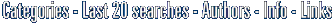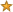(Within our archive we collect a lot of software including: Graphics4VO DLL: Graphics library for CAVO with output to display, printer, file and memory. Rt-Plot: Rt-Plot is a tool to generate Cartesian X/Y-plots from scientific data. Function Grapher: Graph maker to create 2d, 2.5d and 3D function graphs and animations. 3DMath Explorer: 3DMath Explorer - 3D Graph Plotting Software for Math, Science and Engineering. Function Grapher Standard Edition: Create 2D,2.5D, 3D function graphs and animations Graph: Program for drawing graphs of mathematical functions in a coordinate system. Equation Grapher: Equation grapher is an easy-to-use software for 2D function graphing. GraphSight: Feature-rich comprehensive 2D math graphing utility with easy navigation. ; these are very useful for graph function)FindGraph 2.48- Graphing, trend analysis and curve matching software.[ Get it - More information and user's reviews about FindGraph ] (This software is related to: math graph curve fitting equation chart plotting visualize digitizing drawi...)Download FindGraph Graphing, trend analysis and curve matching software.Functions 1.00- Studies functions of one variable to find roots, extrema, integral, graph[ Get it - More information and user's reviews about Functions ] (This software is related to: math mathematics graphics functions graph engineering formula equation scie...)Download Functions Studies functions of one variable to find roots, extrema, integral, graphMathProf 4.0- MathProf is an easy to use mathematics program within approximately 180 subrouti[ Get it - More information and user's reviews about MathProf ] (This software is related to: Mathematics Maths Formula Educationsoftware Teachers Students High school L...)Download MathProf MathProf is an easy to use mathematics program within approximately 180 subroutiVisual Complex 1.6- Visual Complex is a graph software to create graph of complex function. [ Get it - More information and user's reviews about Visual Complex ] (This software is related to: complex grapher graph software complex graphs...)Download Visual Complex Visual Complex is a graph software to create graph of complex function.GraphSight 2.0- Feature-rich comprehensive 2D math graphing utility with easy navigation.[ Get it - More information and user's reviews about GraphSight ] (This software is related to: GraphSight plot graph graphs function functions math mathematics 2D graphic...)Download GraphSight Feature-rich comprehensive 2D math graphing utility with easy navigation.Function Grapher Standard Edition 2.1.0- Create 2D,2.5D, 3D function graphs and animations[ Get it - More information and user's reviews about Function Grapher Standard Edition ] (See also: grapher and function or better equation and cheap graph or graphs and also ...)Download Function Grapher Standard Edition Create 2D,2.5D, 3D function graphs and animations3DMath Explorer 3.1- 3DMath Explorer - 3D Graph Plotting Software for Math, Science and Engineering.[ Get it - More information and user's reviews about 3DMath Explorer ] (This software is related to: 3dmath 3dmath explorer math mathematics plot plotter graph grapher 2D graph...)Download 3DMath Explorer 3DMath Explorer - 3D Graph Plotting Software for Math, Science and Engineering.Function Grapher 3.7.3- Graph maker to create 2d, 2.5d and 3D function graphs and animations.[ Get it - More information and user's reviews about Function Grapher ] (This software is related to: function grapher graph maker GRAPH FUNCTION GRAPH FUNCTIONs...)Download Function Grapher Graph maker to create 2d, 2.5d and 3D function graphs and animations.Rt-Plot 2.0- Rt-Plot is a tool to generate Cartesian X/Y-plots from scientific data.[ Get it - More information and user's reviews about Rt-Plot ] (See also: PlotCartesian program and also PlotFunction program and plotfunction plotcartesian program and PlotterX Y GraphGraphLinear program and cheap plotterx_y_graphgraphlinear plotfunction program and cheap RegressionRegressionNon program...)Download Rt-Plot Rt-Plot is a tool to generate Cartesian X/Y-plots from scientific data. Graphics4VO DLL 11.04- Graphics library for CAVO with output to display, printer, file and memory.[ Get it - More information and user's reviews about Graphics4VO DLL ] (See also: graphics4vo or better graphic and cheap graphics or chart and also presentation, ...)Download Graphics4VO DLL Graphics library for CAVO with output to display, printer, file and memory. Graph 2.6- Program for drawing graphs of mathematical functions in a coordinate system.[ Get it - More information and user's reviews about Graph ] (See also: mathematic or better graphing and cheap drawing or functions and also tangents, ...)Download Graph Program for drawing graphs of mathematical functions in a coordinate system. Equation Grapher 2.0.5- Equation grapher is an easy-to-use software for 2D function graphing.[ Get it - More information and user's reviews about Equation Grapher ] (This software is related to: grapher function equation graph graphs table...)Download Equation Grapher Equation grapher is an easy-to-use software for 2D function graphing. DeadLine 2.11- Solve equations graphically and numerically.[ Get it - More information and user's reviews about DeadLine ] (This software is related to: free equation solver equation solver equation solve roots root finding deri...)Download DeadLine Solve equations graphically and numerically. Advanced Grapher 2.2- Plot and analyze graphs of equations and data[ Get it - More information and user's reviews about Advanced Grapher ] (This software is related to: graph graphs graphing graphing calculator graphing software regression plot...)Download Advanced Grapher Plot and analyze graphs of equations and data Numbers Up! Baggin' The Dragon Windows 1.1- Fun math game for ages 4-15 - covers geometry, measurement, algebra, data.[ Get it - More information and user's reviews about Numbers Up! Baggin' The Dragon Windows ] (See also: measurement software and also algebra software, shape software and space software or better data software and cheap ...)Download Numbers Up! Baggin' The Dragon Windows Fun math game for ages 4-15 - covers geometry, measurement, algebra, data. Numbers Up! Baggin' the Dragon Mac 1.1- Fun math game for ages 4-15 - covers geometry, measurement, algebra, data.[ Get it - More information and user's reviews about Numbers Up! Baggin' the Dragon Mac ] (This software is related to: measurement algebra shape space data geometry mass area volume graphs funct...)Download Numbers Up! Baggin' the Dragon Mac Fun math game for ages 4-15 - covers geometry, measurement, algebra, data.   Keep yourself updated, subscribe to the soft14 Newsletter now! Enter your email here: